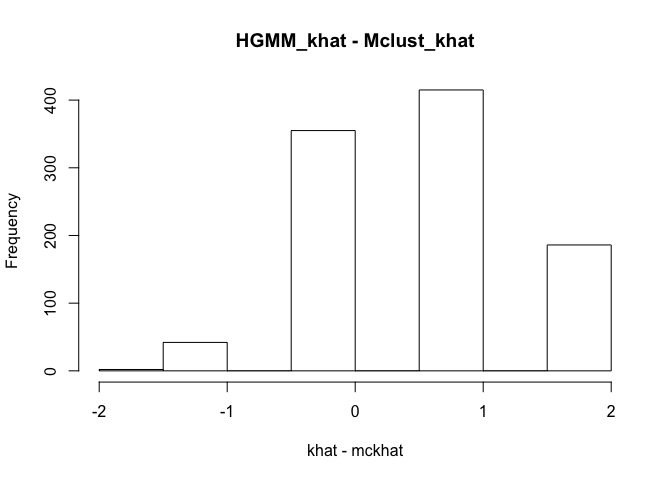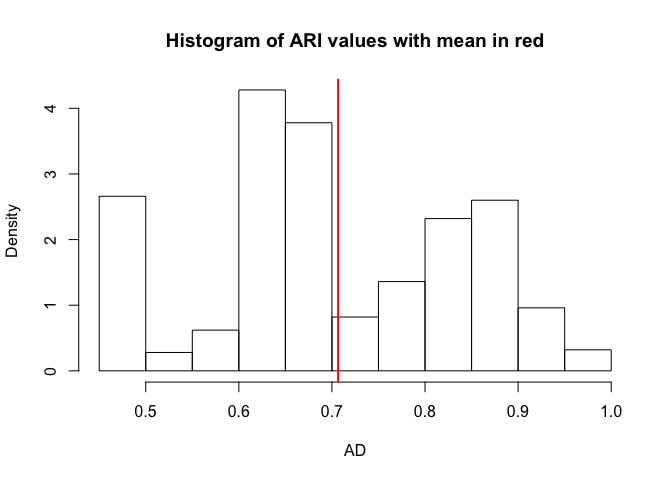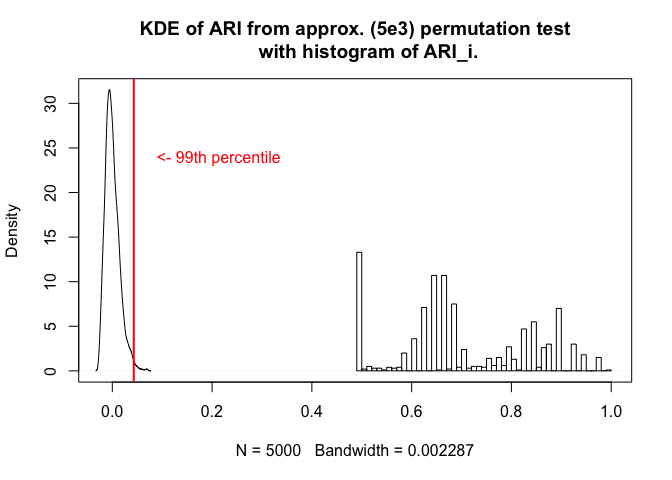mc <- 1000
#set.seed(21218)
#set.seed(317)
set.seed(301)
Y <- foreach(i = 1:mc) %do% {
N <- 100
mu0 <- c(0,1,10,11)
sig20 <- c(0.1,0.1,0.1,0.1)
pi0 <- c(1/4,1/4,1/4,1/4)

#Z <-  apply(rmultinom(N, 1, c(1/4,1/4,1/4,1/4)), 1, sum)
Z <- rep(25,4)

lab <- Reduce(c, mapply(function(x,y) rep(x, each = y),1:4, Z))

tmp <- list()
for(i in 1:length(Z)){
tmp[[i]] <-
rnorm(Z[i], mean = mu0[i], sd = sqrt(sig20[i]))
}

X <- Reduce(c, tmp)
XZ <- data.frame(cbind(X,lab))
list(XZ = XZ, N=N, mu0=mu0, sig20=sig20, pi0=pi0, lab=lab)
}

For each of 1000 Monte Carlo iterates we sample 100 points from a mixture of four Gaussians with means 0, 1, 10, 11 and equal variances of 0.1.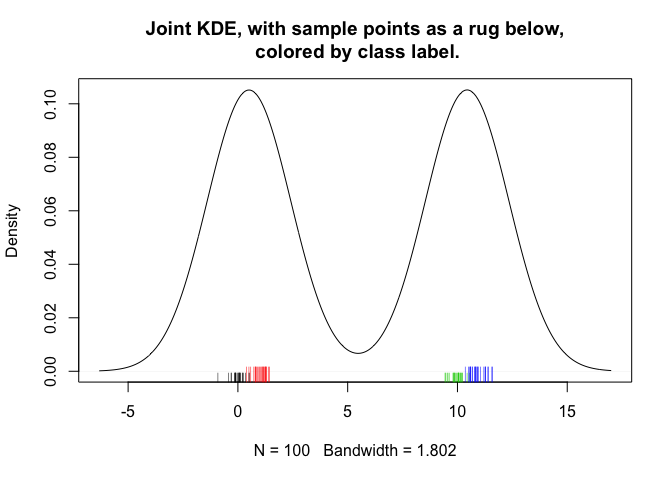##    Min. 1st Qu.  Median    Mean 3rd Qu.    Max.
##    0.00    0.07    0.26    0.22    0.29    0.50
##  0.06855749 0.37136251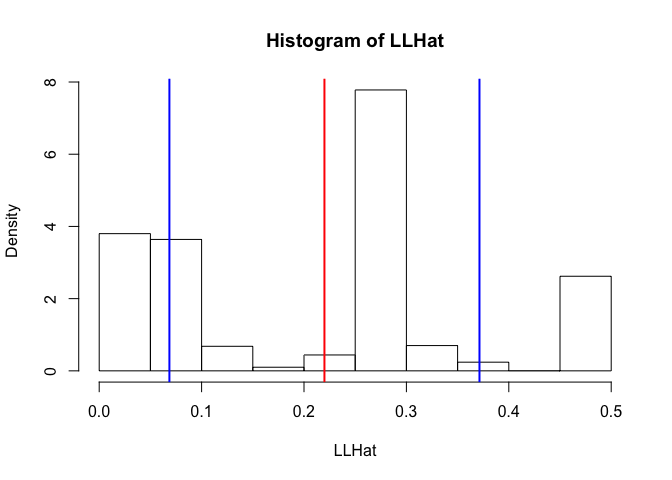## Khat

mckhat <- sapply(mcU, '[[', 6)
#hist(mckhat, main = "Khat from MClust")
kh <- sapply(h, function(x) max(unique(x$dat$labels$col))) kh[which(is.na(kh))] <- 1 khat <- kh KHAT <- data.frame(khat = c(khat, mckhat), cla = rep(c("HGMM", "MCkhat"),each=1e3)) str(KHAT) ## 'data.frame': 2000 obs. of 2 variables: ##$ khat: num  3 2 3 3 3 3 3 4 4 3 ...
##  \$ cla : Factor w/ 2 levels "HGMM","MCkhat": 1 1 1 1 1 1 1 1 1 1 ...
gg1 <- ggplot(dat = KHAT, aes(x = khat, fill = as.factor(cla))) +
geom_histogram(position = "dodge", bins = 10) +
ggtitle("Frequency plot of Khats")

print(gg1)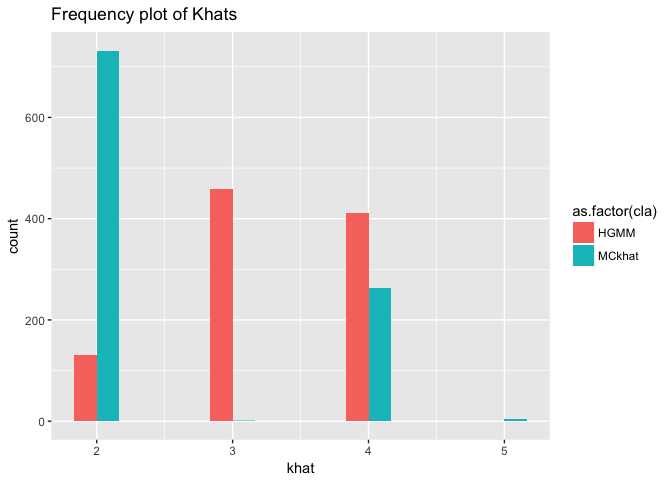## ARI

hist(khat - mckhat, main = "HGMM_khat - Mclust_khat")